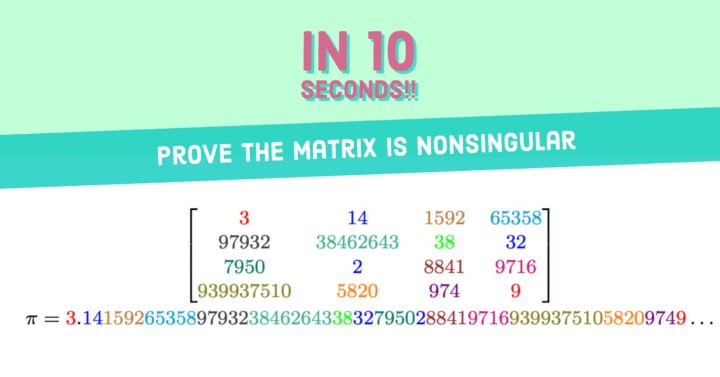# How to Prove a Matrix is Nonsingular in 10 Seconds## Problem 509

Using the numbers appearing in
$\pi=3.1415926535897932384626433832795028841971693993751058209749\dots$ we construct the matrix $A=\begin{bmatrix} 3 & 14 &1592& 65358\\ 97932& 38462643& 38& 32\\ 7950& 2& 8841& 9716\\ 939937510& 5820& 974& 9 \end{bmatrix}.$

Prove that the matrix $A$ is nonsingular.Add to solve later

## Proof.

To show that the matrix $A$ is nonsingular, it suffices to prove that $\det(A)\neq 0$.

One way is to compute the determinant of $A$ directly.

However, as the numbers in $A$ are quite large for hand computation, the direct calculation must be tedious.

So we consider an alternative method.
Note that we do not have to find the exact value of $\det(A)$, but we just need to know $\det(A)\neq 0$.

Thus, it suffices to show that $\det(A)$ is odd. ($0$ is an even number.)
This suggests that considering the matrix modulo $2$ is helpful.

Let $\bar{A}$ be the matrix whose $(i, j)$-entry is the $(i,j)$-entry of $A$ modulo $2$.
That is,
\begin{align*}
\bar{A}:=\begin{bmatrix}
1 & 0 & 0 & 0 \\
0 &1 & 0 & 0 \\
0 & 0 & 1 & 0 \\
0 & 0 & 0 & 1
\end{bmatrix}.
\end{align*}
(Remark that the diagonal entries of $A$ are odd, and off-diagonal entries are even.)

Since $\det(A)$ is a polynomial of entries of $A$, we have
\begin{align*}
\det(A) &\equiv \det(\bar{A}) \pmod{2}\\
&=1.
\end{align*}

It follows that $\det(A)$ is odd, and in particular $\det(A)\neq 0$.
Thus the matrix $A$ is nonsingular.

### What’s $\det(A)$ anyway?

Just for the record, the determinant of $A$ is
$\det(A)=-20330769121541702776233175.$

## Beautiful Formulas for $\pi$

This problem was nothing to do with the number $\pi$ (except we used the digits of $\pi$) and the matrix is far from beautiful.
(Although the method we used is beautiful.)

Check out the post
Beautiful formulas for pi=3.14…
for beautiful formulas containing $\pi$ like

$\pi= \cfrac{4}{1 + \cfrac{1^2}{2 + \cfrac{3^2}{2 + \cfrac{5^2}{2 +\cfrac{7^2}{2 + \cdots}}}}}$Add to solve laterLet $A$ be a square matrix. Prove that the eigenvalues of the transpose $A^{\trans}$ are the same as the eigenvalues...